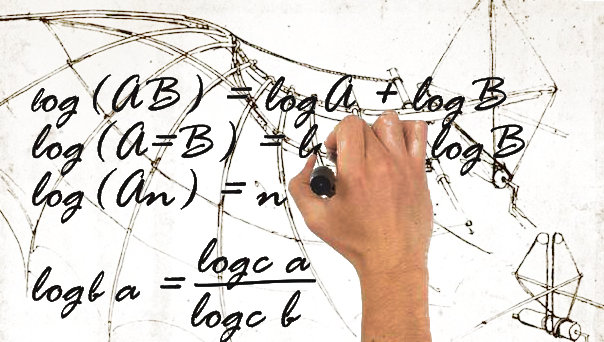## Math Challenge (finished)Thanks Ori for the speedy answer!Whoever gets me the result first in a way I can use it (calculate “percent” based on variable Z and time) can select either a dinner or a 100€ Amazon (or other online available) voucher. We need a curve calculation, fitting the following requirements…

• X-axis is time, Zero (or 30) to infinity
• Y-axis is percent, 0-100
• Variable Z is a positive number between 0 and infinity (real: 1-200 mio).

The curve must not meet the X-Axis, so Y (percent) must always be larger 0, no negative values. At minute 30, the percent value should be 100. Or at minute Zero, then we simply add 30 minutes 😉 Depending on variable Z value, the curve shall slope faster or slower, but “reversed proportional”, the lower Z is, the faster the curve falls. Target values…

• Z=500.000 should fall under 10% at about 60 minutes.
• Z=5.000.000 should fall under 10% at about 90 minutes.
• Z=20.000.000 should fall under 10% at about 120 minutes.
• Z=70.000.000 should fall under 10% at about 180 minutes.

* 10% or 20%, but preferably not more! But the curve bending should be variable based on Z within that rough frame. If we have the curve, we need to calculate the coordinates, so percent (with min. two decimals), at given X minutes. We “know” minutes and the variable Z.

0 - click to show Jürgen you liked the post